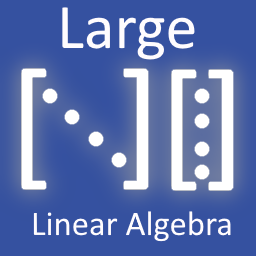#### Large Linear Algebra (c++) 1.0.0Misc4.1Community

Submitted by user Johnnoe; BSL-1.0; 2023-08-29

This GDExtension implements matrix algebra and solving routines for matrices of arbitrary dimensions. It is implemented in c++, using GDExtension to maximise performance. This extension implements three main types, that are directly accessible within GDScript: DenseMatrix, SparseMatrix, VectorN.

Its Features Include:
- Sparse and Dense Matrix representations with double precision arithmetic.
- Efficient Matrix-Matrix and Matrix-Vector multiplication for Dense and Sparse Matrices.
- Heterogenous arithmetic between different Matrix representations and Vectors, including Multiplication, Addition, and Subtraction.
- Routines for the conversion between Dense and Sparse Matrices, and Godot built-in PackedFloat64Array types.
- Both In-place and Not-in-place variants of a number of arithmetical operations, for efficiency. Some operations are in-place only, and Matrices should be explicitly cloned if that is the desired behaviour.
- Direct solving using Gaussian Elimination with Partial Pivoting for Dense Matrices
- Matrix inverses calculated using Gaussian Elimination with Partial Pivoting for Dense Matrices
- Iterative solvers for Dense and Sparse Matrices, using the Conjugate Gradient Method.